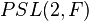# Group having a class-inverting automorphism

Jump to: navigation, search

## Contents

BEWARE! This term is nonstandard and is being used locally within the wiki. [SHOW MORE]
This article defines a group property: a property that can be evaluated to true/false for any given group, invariant under isomorphism
View a complete list of group properties
VIEW RELATED: Group property implications | Group property non-implications |Group metaproperty satisfactions | Group metaproperty dissatisfactions | Group property satisfactions | Group property dissatisfactions

## Definition

A group having a class-inverting automorphism is a group for which there exists a class-inverting automorphism: an automorphism that sends every element to an element in the conjugacy class of its inverse.

## Group families

Group family What groups in the family have a class-inverting automorphism? Proof
alternating group$A_n$$n = 1,2,3,4,5,6,7,8,10,12,14$ Classification of alternating groups having a class-inverting automorphism
symmetric group$S_n$ all$n$ Symmetric groups are rational, rational implies ambivalent (every element is conjugate to its inverse), ambivalent implies there is a class-inverting automorphism (the identity automorphism works)
general linear group$GL(n,F)$ for a natural number$n$ and field$F$ all$n,F$ transpose-inverse map is class-inverting automorphism for general linear group
projective general linear group$PGL(n,F)$ for a natural number$n$ and field$F$ all$n,F$ transpose-inverse map induces class-inverting automorphism on projective general linear group
special linear group of degree two$SL(2,F)$ for a field$F$ all$F$ special linear group of degree two has a class-inverting automorphism
projective special linear group of degree two$PSL(2,F)$ for a field$F$ all$F$ projective special linear group of degree two has a class-inverting automorphism